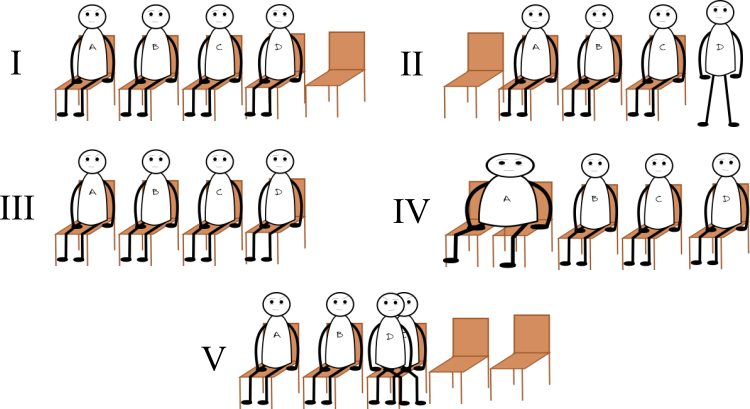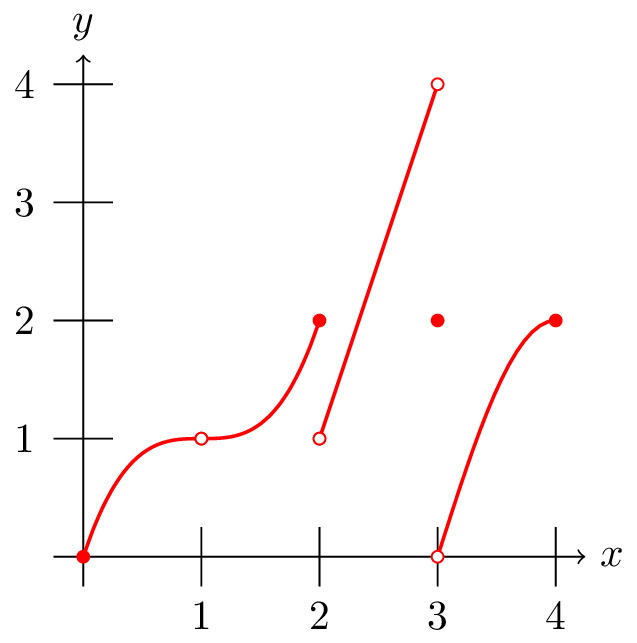# Calculus I

This interactive textbook covers the standard topics for differential calculus .

Functions are the basic building blocks of a lot of mathematics. A function, $f$, is something that takes an item from a set  $A$, messes with it, then spits out one thing from a set $B$.  Every element in  $A$ must be $f$''-able, and f can only spit out stuff that is in $B$

Example 1-1:  Let $A$ be the set of US states and $B$ equal the set of all the cities in the US. We could define a function on $A$ by $f(x)$ is the capital of $x$.  For example, $f(Ohio)=Columbus$.  This is well-defined function since everything in $A$ can be $f$''-ed.

Example 1-2: Again,  let A be the set of US states and B equal the set of all the cities in the US.  If my function were $f(x)$ $= the\, city\, with\, a\, Krispy\, Kreme \,doughnut\, store$, we would have problems. First off, some states, sadly, don't have a Krispy Kreme doughnut store, so the function is not defined on that state. Secondly, some states have many cities with Krispy Kreme doughnut stores, so, say,  $f(North\,\, Carolina)$ has multiple answers.  We could say that this function fails the "vertical" line test at $x=North\,\,Carolina$.

Question 1-1: Sitting in Chairs

Suppose our "function" is f(person) is the chair they are sitting in. In the scenarios below, which ones are NOT functions on the set of people A, B, C, and D:A

I and II

B

II and IV

C

V and IV

D

IV and V

## Some vocabulary

Suppose we have a function, $f$, taking elements from $A$ and spitting out elements from $B$. The standard notation for this is:

$f: A \to B$ .

What goes into a function,  set $A$, is the domain.  What comes out of the function is the range.  Since in a way, we can choose what goes in,  the variable(s) that represent stuff in $A$  is the independent variable. What comes out depends on what went in, so the variable(s) that represent stuff in $B$ is the dependent variable. Notice, in the example above, not every city in $B$ was spit out by $f$.  So, $B$ is not the range.  We call $B$ the codomain. Everything in $B$ that gets spit out by $f$ is called the range. If everything in $B$ gets hit'', then the function is onto. (ON-to, get it?) If $f(x)$ is unique (no 2 $x$'s spit out the same thing), then the function is one-to-one (1-1).

Example 1-3:   In the example above where $f(x)$ is the capital of $x$, the function is NOT onto because not every city is the capital of its state.  The function IS 1-1 since no two states have the same capital.

Question 1-2: Chairs revisited

If we let f(x) = the chair that x sits in, which of the following are 1-1 functions?A

I and III

B

I and II

C

II and III

D

I, II, and III

Question 1-3: More chairs...

Again with the chairs! Which of the following are onto functions?A

III

B

IV

C

III and IV

## Some notation

You've probably seen $f(x)$,  $g(x)$, and maybe even a $h(x)$. Hopefully, you know this does NOT mean to multiply $f$ and $x$. This is read $f$ OF $x$.  We use letters to denote generic functions and functions we define.  There are specific functions with the same format: $sin(x)$, $cos(x)$, $ln(x)$, etc. Since these words are well known functions that can't exist mathematically without their x's,  we sometimes get lazy and drop the parenthesis. The parenthesis is (are?) understood.  Writing just "$sin$" is wrong, a sin, if you will.  Some of the funkier functions, in terms of notation, are the exponentials. There are no paranthesis, and the $x$ is in the air: $e^x$.  Many computer programs will write: $e^x$ as $exp(x)$ so all the functions follow the same format.

When you define a function, $f(x)$, $x$ is just a place holder. When you evaluate $f(something)$ then where ever there was an $x$ in the definition of f(x), you replace the $x$ with the $someting$.  If something actually contains an $x$, don't let that freak you out.  It's not the same as the defining $x$.

Example 1-4: Consider $\;$$f(x) = x^2+5+3\sqrt{x^3-2x+1}$ .

Then we can say:

$f(z)=(z)^2+5+3\sqrt{z^3-2z+1}$

$f(\beta)=(\beta)^2+5+3\sqrt{\beta^3-2\beta+1}$

$f(x+h)=(x+h)^2+5+3\sqrt{(x+h)^3-2(x+h)+1}$This Content is Locked
Only a limited preview of this text is available. You'll need to sign up to Top Hat, and be a verified professor to have full access to view and teach with the content.

## Finding the domain

An important component of a function is what can you put into it? When you are defining a function, you can always specify its domain.  Consider $f(x)=x^2$, with a domain of just  integers:

If the domain is not specified, you assume it is the largest subset of real numbers for which the function is defined.  Typically, the only problems come from when you put a 0 in the denominator of a fraction or try to take the (even) root of a negative number.  (The even root of a negative number is a complex number, which is fine and dandy, but at this level, we try to avoid complex numbers.) When we find the domain, we kick out the numbers that don't work.

Example 1-5: Suppose we have $f(x)=\frac{1}{x}$. The only real number we can't put in there is 0. How do we denote this mathematically. There are several ways:

$D_f = {\mathbb{R}}-\{0\}$

I like this one. Short and sweet, very intuitive.  "The domain of $f$ is the real numbers ( ${\mathbb{R}}$) take away 0." Interval notation also works:

$D_f = (-\infty,0) \bigcup (0,\infty)$

Or, this notation:

$D_f = \{x \in {\mathbb{R}}| x \neq0\}$

but that seems WAY too long to me for this particular domain. Which version is "right"? Whichever one you feel most comfortable with.  I tend to go for the shortest one because I'm lazy.

Example 1-6: Consider $f(x) = \sqrt{x-2}$. This is an even root, so only non-negative terms can be in the domain.  Which means $x\geq 2$.

$D_f = [2,\infty)$

For this example, interval notation worked nicely.

Example 1-7: Consider $f(x) = \frac{x}{\sqrt{x^2-4}}$. When finding the domain, I don't care about the x on top. The interesting part is the $\sqrt{x^2-4}$: the part under the radical (aka radicand...you'll remember that, right?) must be positive. This means that $x>2$ or $x<-2$.  Notationally, we can write:

$D_f=(-\infty,-2) \bigcup (2,\infty).$

We could also write

$D_f = {\mathbb{R}} - [-2,2]$.

Or we could even write

$D_f = \{ x | x > 2\,\, or \,\, x<-2\}$

Example 1-8: Let's try an oogy one: $f(x) = \frac{\sqrt{x+2}}{(x-3)\sqrt{x^2-1}}$.  Don't panic. Take it one chunk at a time, and then we'll find the intersection of what's allowable.

First, $\sqrt{x+2}$:  for this, $x\geq -2$. Since the square root is on top, the stuff under the radical can be $0$ , so we can include the $-2$.

Next, $(x-3)$ in the denominator kicks $3$ out of the domain.

Finally, $\sqrt{x^2-1}$ in the denominator forces $x^2-1 > 0$, so $x>1$ or $x<-1$.  Since this radical is in the denominator, we can't include $\pm 1$ since they would give us 0 in the denominator.

Let's put it all together and see what we get:

Yikes!  Okay, the red is the intersection. So, the domain is $[-2,-1) \cup (1,3) \cup (3,\infty)$.

One more:

Example 1-9: $f(x) = \sqrt{(x-1)(x+1)(x-2)}$.  I went ahead and gave you the factored radicand so we don't get bogged down in factoring at this point.  Our task is to find when $(x-1)(x+1)(x-2)\geq 0$ .  The only places where the expression $(x-1)(x+1)(x-2)$ could change sign is at $1$, $-1$, and $2$.  This gives us four sections.

The plus/minus-ness in each section stays the same, so we need only check a convenient number from each section. I choose $-2$, $0$, $1.5$, and $3$ . I then plugged each of these into the expression. I only simplified enough to see if it was positive or negative. Here's what I got:

Since the expression can't be negative, the domain is $[-1,1]\cup [2,\infty)$This Content is Locked
Only a limited preview of this text is available. You'll need to sign up to Top Hat, and be a verified professor to have full access to view and teach with the content.

## A word on continuity

For the most part , the functions we look at will be continuous on their domain. Continuous...can you draw it without lifting your pencil/pen? There is a MUCH more formal definition which we'll look at later, but intuitively, that's what we mean by continuity.

A function is continuous on the interval $[a,b]$ if it is continuous on $(a,b)$, continuous from the right at $a$ and continuous from the left at $b$.  If a point is not on the graph, then the function cannot be continuous at that point. Let me illustrate with a graph.

Looking at the point $(1,2)$, we can draw that point from the left, but not from the right, so the graph is continuous from the left at $x=1$.  Looking at the largest intervals possible,  the intervals over which this function is continuous are: $[0,1]$,  $(1,2]$, $(2,3)$, $(3,4]$

Question 1-6: Continuity Question

Over which intervals is $f(x)$ not continuous?A

$[0,1)$

B

$(2,3)$

C

$(0,2)$

D

$[1.25,1.75]$

## Combining Functions

We can add, subtract, multiply, and divide these functions to create new functions. As noted above,  polynomials are just products and sums of power functions with non-negative, integer powers. Rational functions are quotients of polynomials.  These combinations are special because we see them a lot and they have common properties, so they get their own names.

All these functions can be added, subtracted, multiplied, and divided.  You treat them as you do numbers: use PEMDAS.  You used these combinations to create polynomials and rational functions.  A funkier operation on functions is composition.  Composition is when you put one function inside of the other function.  In terms of what is happening, the inside function is evaluated first, the outside function is evaluated second. This is denoted by $(f\circ g)(x)=f(g(x))$. Written this way,  $g(x)$ goes first, $f(x)$ goes second. If we write $(g\circ f)(x)=g(f(x))$, then $f(x)$ goes first and $g(x)$ goes second.

Let's do a bunch of examples.

Example 1-10: Let $f(x) = x^2+1$ and $g(x) = \sin (x)$.

$(f\circ g)(x) = f(g(x)) = f(\sin(x))=(\sin(x))^2+1$      (aka $\sin^2(x)+1$

$(g\circ f)(x) = g(f(x))=g(x^2+1)=\sin(x^2+1)$

Notice we got two different answers. When composing functions, order matters. Composition of functions is not commutative.

Example 1-11:  Let $f(x) = \sqrt{x^3+7x-5}$ and $g(x) = 2x+1$.

$(f\circ g)(x) = f(g(x))=f(2x+1)=\sqrt{\left(2x+1\right)^3 + 7(2x+1)-5}$

$(g\circ f)(x) = g(f(x)) = g(\sqrt{x^3+7x-5}) = 2\left( \sqrt{x^3+7x-5}\right) +1$

Example 1-12:  Let $f(x) = \tan(x) \sec(x)$ and $g(x) = \sin(x)+\cos(x)$.

$(f\circ g)(x) = f(g(x)) = f(\sin(x)+\cos(x))$

$= \tan(\sin(x)+\cos(x))\sec(\sin(x)+\cos(x))$

$(g\circ f)(x) = g(f(x))=g(\tan(x)\sec(x))$

$= \sin(\tan(x)\sec(x))+\cos(\tan(x)\sec(x))$

Question 1-7: Composition of Functions

Let $f(x) = 3x^4+2^x$ and $g(x)=\sin(x)$. Find $f\circ g$ and $g\circ f$.

## Image References

All images and equations in this chapter, including those embedded in questions, were generated by the author, Dr. Maegan K. Bos, using Inkscape, LaTeX, and tikz. Handwriting font created by John F. Bos, Jr.

If you don't know what the vertical line test, your previous math teachers should be pummled with a wet noodle. If you have a graph and can draw a vertical line in such at way that is touches the graph in more than one place, then it is not the graph of a function.
Point versus interval
If I just write (2,5), there's no way to know if I mean the point (2,5) or the interval (2,5). Ya gotta just figure it out from context. And (5,2) is definitely NOT an interval since it doesn't go from smallest to largest.